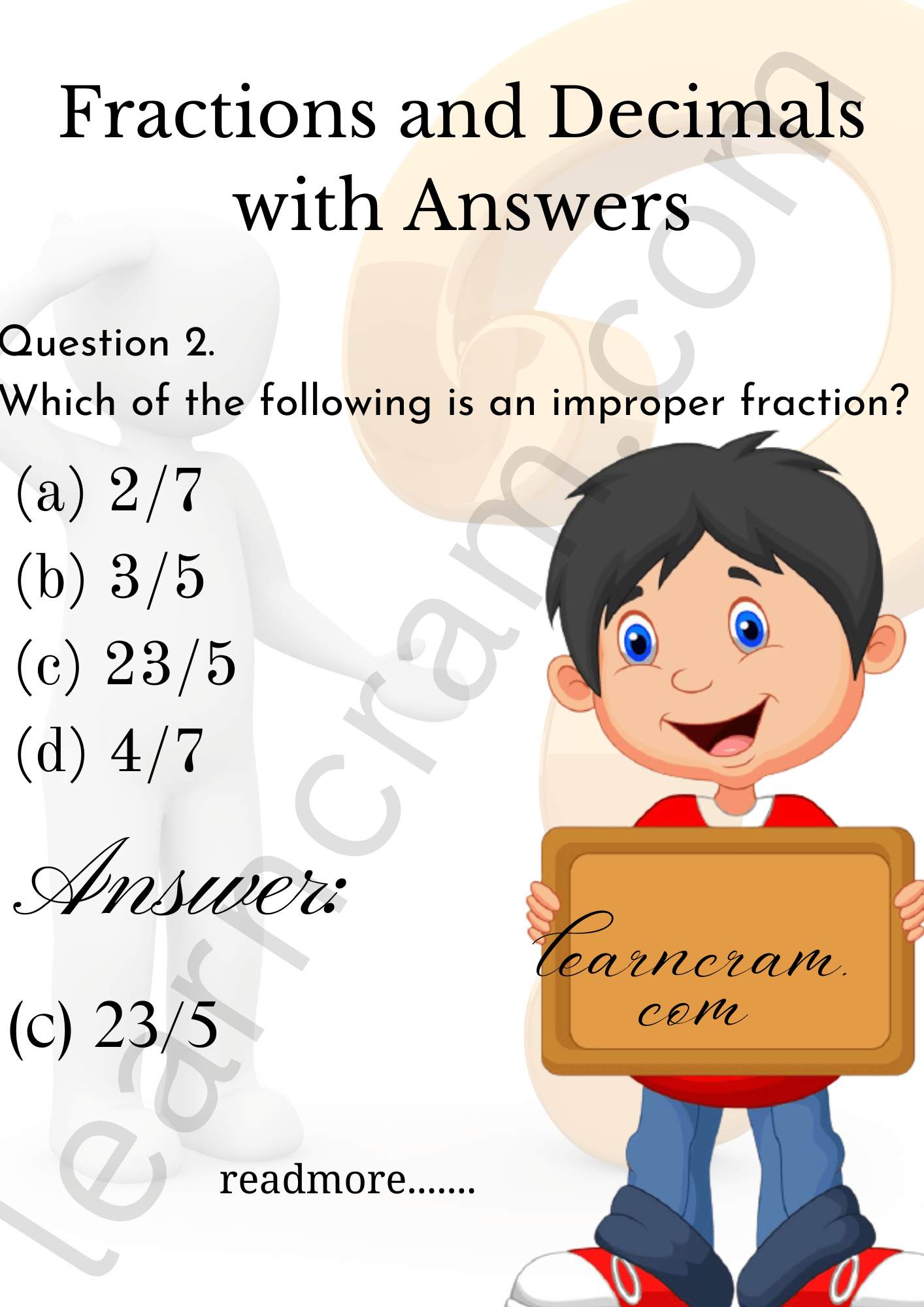# MCQ Questions for Class 7 Maths Chapter 2 Fractions and Decimals with Answers

We have compiled the NCERT MCQ Questions for Class 7 Maths Chapter 2 Fractions and Decimals with Answers Pdf free download covering the entire syllabus. Practice MCQ Questions for Class 7 Maths with Answers on a daily basis and score well in exams. Refer to the Fractions and Decimals Class 7 MCQs Questions with Answers here along with a detailed explanation.

## Fractions and Decimals Class 7 MCQs Questions with Answers

Choose the correct option.

Question 1.
Which of the following is a proper fraction?
(a) $$\frac {5}{3}$$
(b) $$\frac {17}{11}$$
(c) $$\frac {5}{8}$$
(d) $$\frac {13}{9}$$

Answer: (c) $$\frac {5}{8}$$Question 2.
Which of the following is an improper fraction?
(a) $$\frac {2}{7}$$
(b) $$\frac {3}{5}$$
(c) $$\frac {23}{5}$$
(d) $$\frac {4}{7}$$

Answer: (c) $$\frac {23}{5}$$Question 3.
The improper fraction $$\frac {35}{8}$$ in the form of a mixed fraction is.
(a) 4$$\frac {3}{4}$$
(b) 4$$\frac {3}{8}$$
(c) 3$$\frac {7}{8}$$
(d) 4$$\frac {7}{8}$$

Answer: (b) 4$$\frac {3}{8}$$

Question 4.
The value of 1$$\frac {3}{8}$$ is equal to
(a) $$\frac {8}{5}$$
(b) $$\frac {7}{5}$$
(c) $$\frac {9}{5}$$
(d) $$\frac {5}{9}$$

Answer: (a) $$\frac {8}{5}$$

Question 5.
The reciprocal of a proper fraction is
(a) 1
(b) an improper fraction
(c) also a proper fraction
(d) a unit fraction

Question 6.
When the product of two fractions is unity, each is called the
(a) denominator of the other
(b) numerator of the other
(c) additive inverse of the other
(d) reciprocal of the other

Answer: (d) reciprocal of the other

Question 7.
To multiply a decimal number by 100, shift the decimal point to the right by
(a) one place
(b) 2 places
(c) 3 places
(d) 4 places

Question 8.
To divide a decimal number by 1000, shift the decimal point to the left by
(a) one place
(b) 2 places
(c) 3 places
(d) 4 places

Question 9.
7 paise is equal to
(a) Rs 0.7
(b) Rs 0.07
(c) Rs 0.007
(d) Rs 0.0007

Question 10.
How much less is 10 km than 30.6 km?
(a) 10.6 km
(b) 20.6 km
(c) 30.6 km
(d) 10 km

Question 11.
The value of $$\frac {1}{2}$$ × $$\frac {1}{3}$$ × 2 × 3 is
(a) 0
(b) 1
(c) 2
(d) 3

Question 12.
When the product of two fractions is unity, each is called the:
(a) denominator of the other
(b) numerator of the other
(c) additive inverse of the other
(d) reciprocal of the other

Answer: (d) reciprocal of the other

Fill in the blanks

Question 1.
The reciprocal of 2$$\frac {3}{4}$$ is ……………….

Answer: $$\frac {4}{11}$$

Question 2.
5.01 + 6.02 + 8.91= ………………

Question 3.
25 paise is equal to …………….

Question 4.
The improper fraction $$\frac {25}{17}$$ in the form of mixed fraction is ……………..

Answer: 1$$\frac {8}{17}$$

Question 5.
A fraction having its numerator as 1 is called a ………………… fraction, [unit/like]

Question 6.
Fraction having denominator as 10, 100, 1000, etc., are known as ………………… fractions, [unlike/decimal]

Question 7.
$$\frac {6}{11}$$ × …………………. = $$\frac {6}{11}$$

Question 8.
………………. × 1 = 3$$\frac {1}{2}$$

Answer: 3$$\frac {1}{2}$$

Question 9.
The reciprocal of 1 is ……………………

Question 10.
The reciprocal of 0 is …………………..

Question 11.
(Any fraction) ÷ 1 = ……………………

Question 12.
(A whole number) ÷ (A fraction) = (The whole number) × ……………………

Answer: The reciprocal of the fraction

Question 13.
When a decimal is divided by 10, the decimal point moves to the left by ………………… place.

Question 14.
When a decimal is divided by …………………, the decimal point moves to the left by two places.

Match the following

 Column I Column II (a) 3.04 + 2.13 – 0.17 (i) 10 (b) The product of 0 and 2$$\frac {3}{5}$$ (ii) $$\frac {7}{3}$$ (c) The reciprocal of 1 is (iii) 5 (d) The reciprocal of $$\frac {3}{7}$$ is (iv) 0 (e) The value of 18.35 ÷ 1.835 is (v) 1

 Column I Column II (a) 3.04 + 2.13 – 0.17 (iii) 5 (b) The product of 0 and 2$$\frac {3}{5}$$ (iv) 0 (c) The reciprocal of 1 is (v) 1 (d) The reciprocal of $$\frac {3}{7}$$ is (ii) $$\frac {7}{3}$$ (e) The value of 18.35 ÷ 1.835 is (i) 10